U.S. Dept. of Commerce / NOAA / OAR / PMEL / Publications

## Variability of the sea surface temperature in the eastern equatorial Pacific during 1986-88

### (Mixed Layer Temperature Variability, continued)

#### Vertical Entrainment

The vertical turbulent entrainment across the base of the mixed layer can deepen the mixed layer and contributes a term Qto equation (1):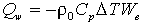(8)

whereT is the temperature jump across the mixed layer base. Estimation of Qrequires a measure of WandT. Following McPhaden , W= W > 0 where(9a)

The time rate of change of mixed layer depth ish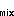/t and W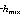is the vertical velocity in the thermocline just below the base of the mixed layer. Requiring W > 0 is necessary to satisfy the condition that entrainment can only cool the mixed layer. Assuming negligible heat flux across isotherms and ignoring horizontal advective terms in the thermocline, the entrainment velocity can be approximated by: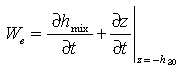(9b)

The depth of the 20°C isotherm (h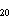) is used to represent thermocline motions, since this isotherm is usually just below the mixed layer in the low-pass-filtered time series (Figure 5).

The appropriate temperature differenceT at the base of the mixed layer is difficult to specify a priori. However, we estimated this temperature by computing the linear regression between -CWand Q. The correlation coefficient was 0.64, and the regression coefficient (1.7°C) was used forT in equation (8). The mean temperature gradient below the mixed layer was about 0.1°C m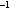, so this average value ofT represents upwelling from about 20 m deeper than h.

Our estimates of WandT were used to compute Q(Figure 6c). This time series is well correlated with the mixed layer heating (correlation coefficient of 0.64 as noted above) and has a standard deviation of 22 W m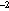. Both positive and negative values of Qare included in Figure 6c and the correlation, to take into account the fact that the local winds are almost always upwelling favorable. Thus one can consider the fluctuations of Qto be superimposed on a mean cooling, with positive values of Qrepresenting a reduction in this cooling.

Variability in Qwas not significantly correlated with the local zonal wind speed. The largest fluctuations in Qwere between early July and mid-September 1986 and between November 1986 and January 1987. In both cases the change in Qwas almost 85 W mand was in phase with the fluctuations of mixed layer heating. Local wind variations during this period were small.

Go back to previous section or forward to next section

PMEL Outstanding Papers

PMEL Publications Search

PMEL Homepage